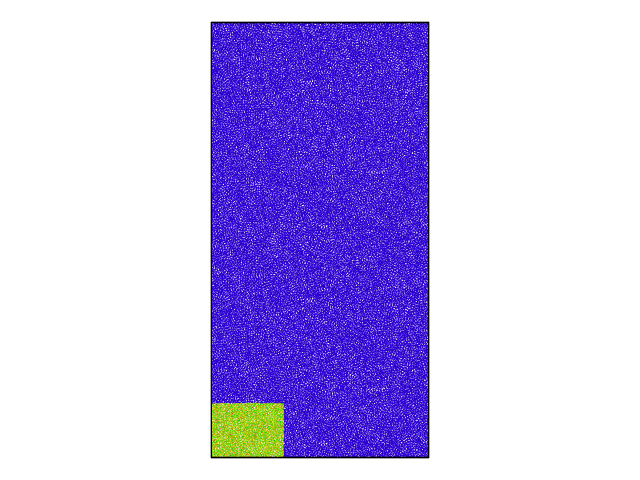# LAMMPS: bug in voronoi analysis of triclinic cells

Dear Steve,
I found a bug in the voronoi analysis (compute_voronoi_atom.cpp) in LAMMPS concerning triclinic cells, where only a part of the simulation cell gets computed. Please find attached a snapshot showing a visualization of the problem, where only the bottom left corner has values for the voronoi volume and everywhere else it is zero. In addition, this is not related to MPI, as even the serial run produces the same problem.
The change got introduced with the 31Oct15 version of LAMMPS and is currently not on the list of known bugs.
I would be grateful if you could have a look into the matter for one of the upcoming releases.
Yours sincerely

Richard JanaHi - please post a small example input script which

shows the problem?

Thanks,

Steve

Hi,
thanks for the fast reply. The attached input script doesn’t do much, but already shows the problem.

Richard

example.in (730 Bytes)

Hi,
thanks for the fast reply. The attached input script doesn't do much, but

​richard,

the attached script uses a custom dump format, that is not part of the
standard lammps distribution. if i change the dump to a custom style dump
and run the input with the current development code, there are no apparent
problems outputting voronoi cell data.

axel.​

Hi,

Following your suggestion we tried all tests using both the “custom” dump style and the “nc” one. They do not seem to influence the results. To reproduce the bug, find attached a simple input file to show this problem clearly. We set a triclinc cell by using “region … prism” this time. Running it we got to something wrong - only a part of the atoms have the values but the others are zero (see the dump output). Again, there was no difference between running the code in serial or parallel running. All calculations mentioned above were performed with the 16Feb16 version of LAMMPS. However, with the 15May 15 version the problem never turns up (not with “prism”, in parallel, or nc dump style). So the bug seems to be in new version. Hopefully the representation of our problem is clearer this time.

Richard

example.in (607 Bytes)

dump.0 (22.3 KB)

Hi,

Following your suggestion we tried all tests using both the "custom" dump
style and the "nc" one. They do not seem to influence the results. To
reproduce the bug, find attached a simple input file to show this problem
clearly. We set a triclinc cell by using "region ... prism" this time.
Running it we got to something wrong - only a part of the atoms have the
values but the others are zero (see the dump output). Again, there was no
difference between running the code in serial or parallel running. All
calculations mentioned above were performed with the 16Feb16 version of
LAMMPS. However, with the 15May 15 version the problem never turns up (not
with "prism", in parallel, or nc dump style). So the bug seems to be in new
version. Hopefully the representation of our problem is clearer this time.

​thanks. i can reproduce the issue, and after some poking around in the
code and some guessing, i came up with the following ​change, which seems
to resolve the issue for the test case. please give it a try and let us
know.

axel.

diff --git a/src/VORONOI/compute_voronoi_atom.cpp
b/src/VORONOI/compute_voronoi_atom.cpp
index b349cbd..4278f12 100644
--- a/src/VORONOI/compute_voronoi_atom.cpp
+++ b/src/VORONOI/compute_voronoi_atom.cpp
@@ -247,7 +247,7 @@ void ComputeVoronoi::buildCells()
for (i = 0; i < nlocal; i++) voro[i] = voro[i] = voro[i] =
0.0;
}

- double *sublo = domain->sublo, *sublo_lamda = domain->sublo_lamda,
*boxlo = domain->boxlo;
+ double *sublo = domain->sublo, *sublo_lamda = domain->sublo_lamda,
*boxlo = domain->boxlo, *boxhi = domain->boxhi;
double *subhi = domain->subhi, *subhi_lamda = domain->subhi_lamda;
double *cut = comm->cutghost;
double sublo_bound, subhi_bound, cut_bound;
@@ -274,9 +274,9 @@ void ComputeVoronoi::buildCells()
sublo_bound = h*sublo_bound + h*sublo_bound +
h*sublo_bound + boxlo;
sublo_bound = h*sublo_bound + h*sublo_bound + boxlo;
sublo_bound = h*sublo_bound + boxlo;
- subhi_bound = h*subhi_bound + h*subhi_bound +
h*subhi_bound + boxlo;
- subhi_bound = h*subhi_bound + h*subhi_bound + boxlo;
- subhi_bound = h*subhi_bound + boxlo;
+ subhi_bound = h*subhi_bound + h*subhi_bound +
h*subhi_bound + boxhi;
+ subhi_bound = h*subhi_bound + h*subhi_bound + boxhi;
+ subhi_bound = h*subhi_bound + boxhi;

} else {
// orthogonal box

I can see why that would eliminate the problem, but it is not correct. I tracked the problem down to a change I made in January. I just checked in corrected compute_voronoi_atom.cpp (marked by + below):

// cutghost is in lamda coordinates for triclinic boxes, use subxx_lamda
double *h = domain->h;
for( i=0; i<3; ++i ) {

• sublo_bound[i] = sublo[i]-cut[i]-e;
• subhi_bound[i] = subhi[i]+cut[i]+e;
• sublo_bound[i] = sublo_lamda[i]-cut[i]-e;
• subhi_bound[i] = subhi_lamda[i]+cut[i]+e;

I can see why that would eliminate the problem, but it is not correct. I
tracked the problem down to a change I made in January. I just checked in
corrected compute_voronoi_atom.cpp (marked by + below):

​thanks aidan!

it is always better to have patches from people who _know_ (what they are
doing), than from people who _guess​_ (badly).axel.